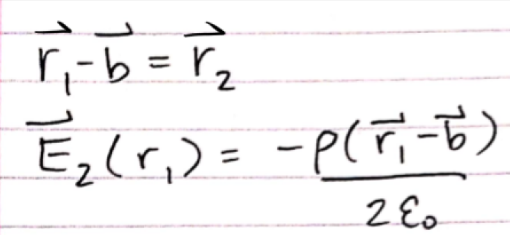# Electrostatics: Gauss's Law & Superposition Principle

Gauss’s Law is a useful tool for solving electrostatics problems. Instead of computing hard nasty integrals, we can use Gauss’s Law to simplify the computation for nice symmetric cases.## Gaussian Surfaces

To start, we’ll define a Gaussian surface. A Gaussian surface is a CLOSED surface that contains some electric charge. For instance, below we have an electron represented by the minus sign and a Gaussian surface represented by dotted lines.The Gaussian surface isn’t necessarily geometrically constricted; however, choosing highly symmetric surfaces helps with problem solving. If the problem scenario is the same from all angles (i.e. no matter which angle you look at the problem, it is the same) spherical coordinates and spherical Gaussian surfaces can help reduce problem complexity. If the problem scenario has circular symmetry along an axis, cylindrical coordinates and surfaces can help.

## Superposition Principle

The superposition principle claims that, in a linear system, the net response of two or more stimuli is the sum of the responses from those stimuli individually.## Example 1: (Warmup)

A solid insulating sphere with charge +q and radius a is surrounded by a conducting shell of unknown charge and radius b where b > a. The electric field outside the shell is 0. Find the electric field inside the shell and the charge density of the shell. Assume the +q charge is uniformly distributed on the volume of the sphere.First, we’ll start with the easier part of the problem. We can tell that the problem has spherical symmetry (they are uniform spheres after all). Therefore, we can draw a spherical Gaussian surface of radius r > b .We also know the electric field outside the shell is 0 as given by the problem statement (how convenient…). Therefore, the integral becomes:And we can solve for the charge density as shown above.

Next, we want to find the electric field inside the conducting shell. We start by drawing another spherical surface inside the shell but outside the sphere of charge:We realize that the charge enclosed by the surface is always +q for b > r > a and the electric field is obtained above. Notice that it is similar to the field generated by a point charge (not too significant for most applications, but the result suggests that point charges can be approximately obtained by using small spheres of charge).

Finally, we want to find the electric field inside the sphere of charge (that’s also inside the shell). We start by drawing yet another spherical surface inside the sphere of charge. We also need to quickly calculate the charge density of the sphere by dividing the charge by the volume of the sphere. This is necessary because unlike the previous cases, the entire sphere isn’t enclosed by the Gaussian surface; therefore, the enclosed charge will depend on how far we are from the center of the sphere of charge.## Example 2: (The Harder One)(The hint is the superposition principle.)

As you can tell, the hole makes the problem a bit more difficult, but it’s nothing we can’t handle. We should be able to find the electric field generated by a solid cylinder with uniform charge density (hopefully) so we want to try to simplify our problem by using the superposition principle to turn the problem into simple cylinders. If the hole has 0 charge, we can just add a large cylinder of charge density ρ and small cylinder – ρ (Think of a neutral atom. It has protons and electrons that each have their individual charges but add up to 0 overall). Since the cylinders have different central axes, we denote them with different subscripts as shown below.We want to find the electric field inside the hole, so we begin by drawing the larger cylinder and an appropriate Gaussian surface.Using Gauss’s Law and working through the integral we find the electric field is:Also, since we are interested in the region inside the hole, which is the region inside both cylinders, we only need to calculate one integral because the small cylinder will have a similar integral, just with a negative sign because of the charge density and a different radius variable.

Next, we want to relate the position vectors so we can add them together. We know that the center of the hole is offset by a distance, b, so we find the following:And finally, adding the electric fields, we obtain the following:Which is a constant.

## Summary

So, in this article, we covered the usage of Gauss’s Law in spherically and cylindrically symmetric cases. We also covered the superposition principle and how to apply it to slightly more complicated charge distributions.## Jia Mao

January 11, 2020

View Profile

#### Get in Touch

Thanks! We'll reach out shortly.